## [W-MA-76] Material Modeling Method for Electromagnetic Field/Iron Loss Analyses Considering Residual Strain

Contents
1. Introduction
2. Proposed method
3. Applying to motors
4. Summary
5. References

### 1. Introduction

Improvement in the accuracy of loss analysis is becoming an important topic in electromagnetic analysis, as the electromagnetic device is aiming for higher efficiency. For example, high-precision material models, such as play model and homogenization method, are increasingly applied to iron loss analyses lately. On the other hand, regarding the influence of residual strain and stress, especially the increase in iron loss, a practical material modeling technique has not been established despite ongoing research.
This paper proposes an electromagnetic field analysis and iron loss analysis based on the assumption that the effect of punching can be expressed as a function of the distance from the cutting surface.

### 2. Proposed Method

Fig. 1 shows the flowchart starting from the material measurement to the FEA analysis. The process consists of single sheet testing, identification of degradation characteristic distribution, and applying the conditions to the FEA model.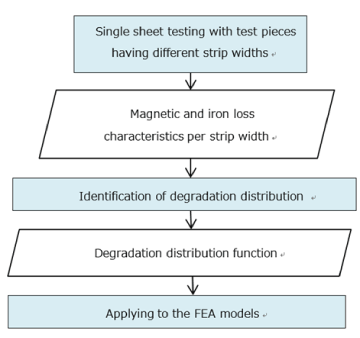Fig. 1 Process of electromagnetic field analysis and iron loss analysis considering the residual strain

To identify the degradation distribution function, magnetic & iron loss characteristics will be measured using non-degradation, and by conducting single sheet testing with different strip widths. Next, degradation distribution is identified by inputting the measured data. Degradation distribution is expressed by multiplying the function form that defines the spatial distribution of degradation by non-degradation characteristics, which is considered the basis. As a result of the identification, the parameters of the functional form are to be obtained. Here, since parameters of the functional form differ depending on the material properties and the method of machining, material property measurement will be necessary, every time the different types of steel and the method of machining are employed. An exponential function is adopted as a functional form. This is based on the assumption that the effect of cutting is the largest at the cutting surface and becomes smaller as it goes inside the material.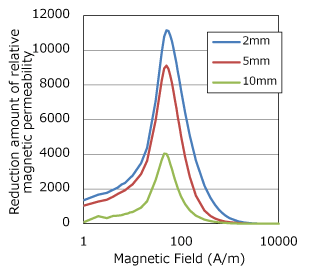(a) Reduction amount of relative magnetic permeability (measured)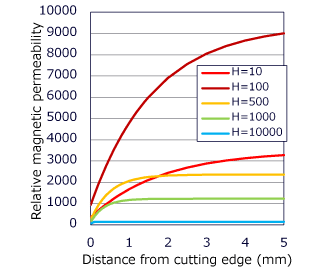(b) Identified relative magnetic permeability distribution

Identifying the degradation distribution function of the magnetic characteristics
Identify the parameters of the relative magnetic permeability distribution function in equation so as to make it fit to the reduction amount of the relative magnetic permeability measured for each cutting width.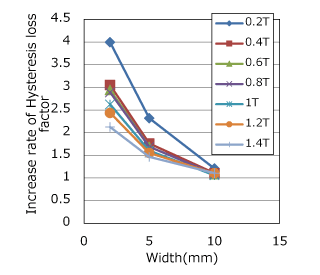(a) Increase rate of hysteresis loss factor (measured)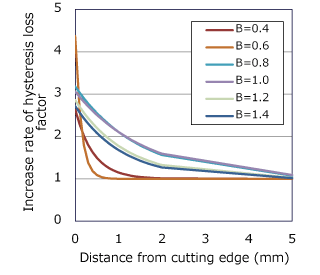(b) Identified hysteresis loss factor increase rate distribution

Identifying the degradation distribution function of the iron loss characteristics
Expresses the degradation of iron loss characteristics by increasing hysteresis loss factor. Identify the parameters of the hysteresis loss factor distribution function in equation so as to make it fit to the hysteresis loss factor increase rate measured for each cutting width.

Protected content here, for members only.
You need to sign in as a JMAG software regular user (paid membership) or JMAG WEB MEMBER (free membership).

By registering as a JMAG WEB MEMBER, you can browse technical materials and other member-only contents for free.
If you are not registered, click the “Create an Account” button.

Remember me